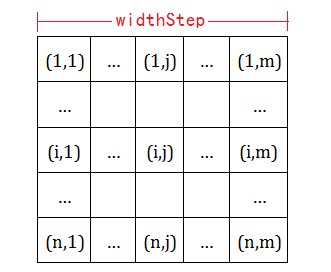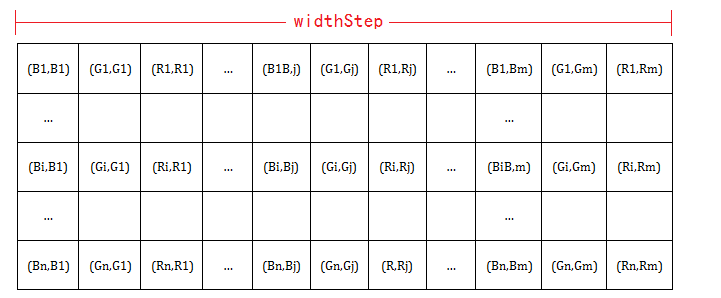#### OpenCV访问图像中每个像素的值

Home / C++ 2016-10-27 2785

IplImage是OpenCV中CxCore部分基础的数据结构，用来表示图像，其中Ipl是Intel Image Processing Library的简写。以下是IplImage的结构分析（来自OpenCV中文网站：http://www.opencv.org.cn/index.php/Cxcore%E5%9F%BA%E7%A1%80%E7%BB%93%E6%9E%84#IplImage
```typedef struct _IplImage
{
int  nSize;         /* IplImage大小 */
int  ID;            /* 版本 (=0)*/
int  nChannels;     /* 大多数OPENCV函数支持1,2,3 或 4 个通道 */
int  alphaChannel;  /* 被OpenCV忽略 */
int  depth;         /* 像素的位深度: IPL_DEPTH_8U, IPL_DEPTH_8S, IPL_DEPTH_16U,
IPL_DEPTH_16S, IPL_DEPTH_32S, IPL_DEPTH_32F and IPL_DEPTH_64F 可支持 */
char colorModel; /* 被OpenCV忽略 */
char channelSeq; /* 同上 */
int  dataOrder;     /* 0 - 交叉存取颜色通道, 1 - 分开的颜色通道.
cvCreateImage只能创建交叉存取图像 */
int  origin;        /* 0 - 顶—左结构,
1 - 底—左结构 (Windows bitmaps 风格) */
int  align;         /* 图像行排列 (4 or 8). OpenCV 忽略它，使用 widthStep 代替 */
int  width;         /* 图像宽像素数 */
int  height;        /* 图像高像素数*/
struct _IplROI *roi;/* 图像感兴趣区域. 当该值非空只对该区域进行处理 */
struct _IplImage *maskROI; /* 在 OpenCV中必须置NULL */
void  *imageId;     /* 同上*/
struct _IplTileInfo *tileInfo; /*同上*/
int  imageSize;     /* 图像数据大小(在交叉存取格式下imageSize=image->height*image->widthStep），单位字节*/
char *imageData;  /* 指向排列的图像数据 */
int  widthStep;   /* 排列的图像行大小，以字节为单位 */
int  BorderMode; /* 边际结束模式, 被OpenCV忽略 */
int  BorderConst; /* 同上 */
char *imageDataOrigin; /* 指针指向一个不同的图像数据结构（不是必须排列的），是为了纠正图像内存分配准备的 */
}
IplImage;```

### 直接访问：```IplImage* img=cvCreateImage(cvSize(640,480),IPL_DEPTH_8U,1);
uchar* tmp;
for(int i=0;i<img->height;i++)
for(int j=0;j<img->width;j++)
*tmp=((uchar *)(img->imageData + i*img->widthStep))[j];```

```IplImage* img=cvCreateImage(cvSize(640,480),IPL_DEPTH_8U,1);
ucha* data=(uchar *)img->imageData;
int step = img->widthStep/sizeof(uchar);
uchar* tmp;
for(int i=0;i<img->height;i++)
for(int j=0;j<img->width;j++)
*tmp=data[i*step+j]; ``````IplImage* img=cvCreateImage(cvSize(640,480),IPL_DEPTH_8U,1);
IplImage* img=cvCreateImage(cvSize(640,480),IPL_DEPTH_8U,3);
uchar* data=(uchar *)img->imageData;
int step = img->widthStep/sizeof(uchar);
int channels = img->nChannels;
uchar *b,*g,*r;
for(int i=0;i<img->height;i++)
for(int j=0;j<img->width;j++){
*b=data[i*step+j*chanels+0];
*g=data[i*step+j*chanels+1];
*r=data[i*step+j*chanels+2];
}  ```

*如果要修改某像素值，则直接赋值。

### 使用cvGet2D()函数访问：

cvGet*D系列函数可以用来返回特定位置的数组元素（一般使用cvGet2D），原型如下：
```CvScalar cvGet1D( const CvArr* arr, int idx0 );
CvScalar cvGet2D( const CvArr* arr, int idx0, int idx1 );
CvScalar cvGet3D( const CvArr* arr, int idx0, int idx1, int idx2 );
CvScalar cvGetND( const CvArr* arr, int* idx );  ```

idx0,idx1,idx2分别用来指示元素数组下标，即cvGet2D返回（idx0,idx1）处元素的值。 因此，单通道图像像素访问方式如下：
```IplImage* img=cvCreateImage(cvSize(640,480),IPL_DEPTH_8U,1);
double tmp;
for(int i=0;i<img->height;i++)
for(int j=0;j<img->width;j++)
tmp=cvGet2D(img,i,j).val;  ```

```IplImage* img=cvCreateImage(cvSize(640,480),IPL_DEPTH_32F,3);
double tmpb,tmpg,bmpr;
for(int i=0;i<img->height;i++)
for(int j=0;j<img->width;j++){
tmpb=cvGet2D(img,i,j).val;
tmpg=cvGet2D(img,i,j).val;
tmpr=cvGet2D(img,i,j).val;
}  ```

```void cvSet1D( CvArr* arr, int idx0, CvScalar value );
void cvSet2D( CvArr* arr, int idx0, int idx1, CvScalar value );
void cvSet3D( CvArr* arr, int idx0, int idx1, int idx2, CvScalar value );
void cvSetND( CvArr* arr, int* idx, CvScalar value ); ```